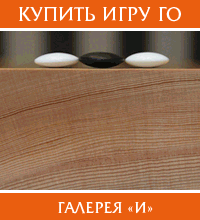Правила Го, задачи для начинающих, партии мастеров Го.
Подфорум курирует А. Динерштейн

18th Chinese Tianyuan titlematch #2 с комментариямиbreakfast на rugo.ru Эксперт Го  +    0    –

Раздел "Лучшая партия месяца" в ИГвР временно (надеюсь) прекращаю вести. Мы решили выбирать самую интересную партию на сайте Go4Go.
Здесь первая из них. Рекомендую! Дух захватывает!

This is a very exciting game. Alexandre Dinerchtein 1p has commented
it and asked me to share it with the Go community. We will start a
special topic on the Go4Go.net website called 'the most exciting game
of the month'. One monthly free commented game will be offered in that
[www.go4go.net] once a month from
now on for more commented games. Please recommend to us if you think a
game is exciting.

(;
GMFFVW[]AP[Many Faces of Go:10.0]
SZ
HA
ST
PB[Gu Li]
PW[Xie He]
DT[2004-03-31]
KM[7.5]
RE[B+3.5]
SO[http://www.go4go.net]
EV[18th Chinese Tianyuan]
BR[7d]
WR[5d]
RO[titlematch#2]
;B[qd]
C[This is the second game from the 18th Chinese Tianyuan titlematch.
Black is Gu Li, 7-dan. White is Xie He, 5-dan
];W[dd];B[pq];W[dp];B[fc];W[cf];B[kc]
C[Gu Li's favourite opening
];W[qk]
(;B[qm]
C[Nowadays extension at X is more popular -
]MA[qn]
;W[qh];B[qf];W[oh]
C[White can defend the group at X as well. Not easy to say, which
move is better
]MA[ok]
;B[pi];W[ph]
(;B[rj]
C[The most agressive plan -
]
(;W[oc]
C[An overplay -
];B[od];W[nd];B[oe];W[mc];B[pc];W[rk];B[ri];W[pj]
;B[rh];W[qo]
C[Too dangerous. X is the only move
]MA[oi]
;B[oi];W[ic];B[ni]
C[The marked stones are huge
]TR[qh][ph][oh]
;W[rm];B[ld];W[ec];B[fd];W[ie]
;B[fg];W[lf];B[kf]
C[Too agressive! It is probably better to defend solidly at X
]MA[ne]
;W[pb];B[qb];W[gf];B[ff];W[ke];B[le];W[kd]
;B[lc];W[mf];B[ne];W[kg];B[jf];W[ig]
C[It seems, that Gu Li, 7-dan missed this move
];B[df];W[ce];B[cg];W[bg]
;B[ch];W[bh];B[ci];W[ng];B[nh];W[og];B[qi];W[jc]
C[The result is clearly favourable for White. The marked stones
cannot escape anymore
]TR[kf][jf]
;B[fq];W[cn]
;B[qp];W[ro];B[np];W[eq]
C[White can defend the corner at X as well
]MA[dr]
;B[fp];W[jq];B[ql];W[rl];B[om];W[rq]
;B[qn];W[rn];B[rp];W[sp];B[qr];W[po];B[nn];W[rr];B[jo]
C[A good move
];W[hp]
;B[ho];W[go];B[ip];W[hq];B[iq];W[ir];B[jr];W[gr];B[is];W[[img]hr[img]]
;B[kq]
(;W[op]
C[The timing is important -
];B[fr]
C[Black has to play actively
];W[hn];B[io];W[er];B[es];W[fo];B[ep];W[do]
;B[gp];W[kr];B[jp];W[gs];B[dq];W[dr];B[cr];W[cq];B[ds];W[dq]
;B[fs];W[br];B[gq]
C[The result is equal
]
(;W[nq]
C[The plan is dangerous -
];B[oq];W[mp];B[no];W[mq];B[or];W[ps];B[nr];W[mr]
;B[ms]
C[The vital point
];W[ls];B[ns];W[lr];B[mo];W[lp];B[lo];W[qq];B[kp]
C[White loses a lot of points here
];W[lb]
;B[kb];W[me];B[md];W[nb];B[nc];W[pp];B[lg]
C[A bad exchange for Black
];W[nd];B[mg];W[nf]
;B[nc];W[kh];B[li];W[if];B[lq];W[nd];B[la];W[mb];B[nc];W[pr]
;B[ks];W[nd];B[qg]
(;W[je]
C[White can also think about atari at X -
]MA[jb]
;B[nc];W[eh];B[eg];W[nd];B[of];W[jg];B[nc];W[ee]
;B[de];W[ed];B[fe];W[cd];B[kf];W[jf];B[pg]
C[An important timing
];W[kf]
C[White's shape is "pretty"
];B[nd]
C[It is better to capture the stone
];W[ej]
;B[gh];W[eo];B[hs];W[dh]
];B[dg];W[hi];B[ei]
C[It seems, that Xie He, 5-dan missed this move
];W[di];B[dj];W[fi]
;B[fh];W[ei];B[gi];W[ek]
C[White has no other way
];B[hj];W[ij];B[ii];W[hh];B[gj];W[ik]
;B[ji];W[ki];B[jj];W[il];B[gb];W[hb];B[eb];W[db];B[hc];W[hd]
;B[ib]
C[A tesuji
];W[ha];B[gc];W[jb];B[ga];W[ia];B[da];W[ca];B[ea];W[fb]
;B[fa];W[bi];B[cb];W[bb];B[ba];W[bc];B[ge]
(;W[he]
C[This is the losing move -
];B[ja];W[ka];B[hg];W[ma];B[el];W[ih];B[dk];W[fk]
;B[gl];W[fl];B[fm];W[em];B[dl];W[gm];B[bj]
C[White loses a lot of points here
];W[bl];B[hm];W[fn]
;B[hl];W[im];B[in];W[kk];B[gn];W[fm];B[ob];W[hn];B[kl];W[kj]
;B[gn];W[cs];B[bk];W[cl];B[ai];W[be];B[al];W[bm];B[dc];W[cc]
;B[hn]
C[The game is over. Gu Li, 7-dan was really lucky!
];W[km];B[ll];W[jm];B[ok];W[pl];B[on];W[pk];B[dm];W[lm]
;B[mm];W[lj];B[lh];W[ml];B[mk];W[nl];B[lk];W[mi];B[mh];W[mj]
;B[nk];W[ol];B[sj];W[sk];B[oa];W[ja];B[dn];W[am];B[cm];W[bn]
;B[ah];W[ag];B[aj];W[kn];B[db];W[ab];B[ak])
(;W[cj];B[hg];W[ih];B[hf];W[lj]
C[White would easily win the game
]))
(;W[jb];B[nc];W[eo];B[of];W[cs]
C[The position would be close
]))
(;W[oq];B[or];W[nq];B[mr];W[mq]
C[This is enough for White
]))
(;W[hn];B[rs];W[qq];B[pp];W[pr];B[ps]
C[White must be afraid of this ko fight
]))
(;W[rk];B[ri];W[pj];B[rh];W[oi]
C[First of all White has to connect the stones
]))
(;B[nd];W[ok];B[po];W[jq]
C[This is the peaceful way
]))
(;B[qn];W[qh];B[qf];W[oh]
C[This is the classic opening
]))

Александр Динерштейн,
Go lessons [breakfast.go4go.net]
Разобранные партии [www.go4go.net]
Журнал "Гоама" [gogame.info]
Новости с серверов Го [GoSensations.com]

Re: 18th Chinese Tianyuan titlematch #2 с комментариямиСаянов Михаил на rugo.ru Любитель Го  +    0    –

Саша, спасибо огромное !

Но что со 180 ходом ?
Почему не были взяты камни ?! ^_^
Или у меня криво отоброзился файл ...

Re: 18th Chinese Tianyuan titlematch #2 с комментариямиСергей Павлов на rugo.ru Знаток Го  +    0    –

Там пропали какие-то символы, по-моему, на границе страниц - из-за отстрочки.

Re: 18th Chinese Tianyuan titlematch #2 с комментариямиPuzzleMan на rugo.ru Любитель Го  +    0    –

Там вместо горизонтальной линии надо вставить W[[img]hr[img]]. Я подправил Сашин пост...

С уважением,
Дмитрий Петраков aka PuzzleMan
Elite Japan Crossword

Re: 18th Chinese Tianyuan titlematch #2 с комментариямиСергей Павлов на rugo.ru Знаток Го  +    0    –

А-а-а... Ну все понятно.

Re: 18th Chinese Tianyuan titlematch #2 с комментариямиСаянов Михаил на rugo.ru Любитель Го  +    0    –

Спасибо, Дима ! =)

Извините, только зарегистрированные пользователи могут писать в этом форуме.

Путь Го       Го-портал       Новости Го